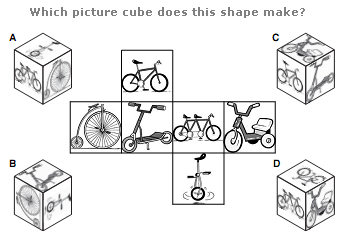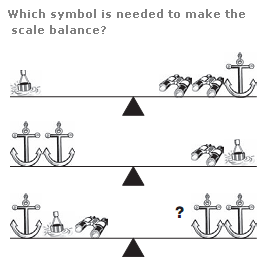# Puzzles - Logical puzzles

### Exercise :: Logical puzzlesAnswer : B Explanation : [NIL]Answer : 4 Explanation : Split the left and right hand circles in half vertically. The letter with the numerical value of the sum of the digits in the left half of the left hand circle is placed in the top left segment of the central circle, and the letter with the numerical value of the sum of the digits in the right half of the left hand circle is placed in the top right segment of the central circle. Repeat this formula for the 2 halves of the right hand circle, putting the resulting letters in the lower segments of the central circle.Answer : Anchor Explanation : The Binoculars = 1, the Anchor = 3 and the Buoy = 5